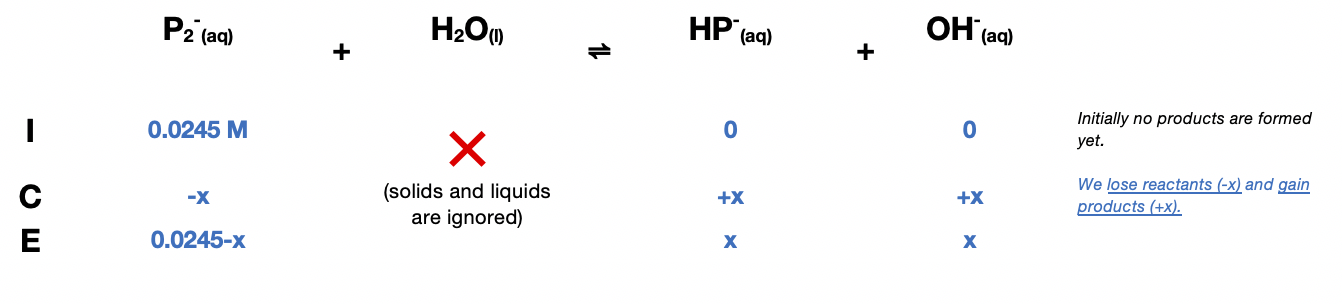# Problem: Potassium hydrogen phthalate, known as KHP (molar mass = 204.22 g/mol), can be obtained in high purity and is used to determine the concentration of solutions of strong bases by the reactionHP- (aq) + OH-(aq) → H2O(l) + P2-(aq)If a typical titration experiment begins with approximately 0.5 g KHP and has a final volume of about 100 mL, what is an appropriate indicator to use? The pKa for HP - is 5.51.

###### FREE Expert Solution

0.0245 M

${\mathbf{K}}_{\mathbf{a}}\mathbf{=}{\mathbf{10}}^{\mathbf{-}{\mathbf{pK}}_{\mathbf{a}}}\mathbf{=}{\mathbf{10}}^{\mathbf{-}\mathbf{5}\mathbf{.}\mathbf{51}}\mathbf{=}$3.09×10-6

${\mathbf{K}}_{\mathbf{b}}\mathbf{=}\frac{{\mathbf{K}}_{\mathbf{w}}}{{\mathbf{K}}_{\mathbf{a}}}\mathbf{=}\frac{\mathbf{1}\mathbf{×}{\mathbf{10}}^{\mathbf{-}\mathbf{14}}}{\mathbf{3}\mathbf{.}\mathbf{09}\mathbf{×}{\mathbf{10}}^{\mathbf{-}\mathbf{6}}}\mathbf{=}$3.24×10-9

Reaction: HP(aq) + OH-(aq) → H2O(l) + P2-(aq)

Construct an ICE Chart:$\overline{){{\mathbf{K}}}_{{\mathbf{b}}}{\mathbf{=}}\frac{\mathbf{products}}{\mathbf{reactants}}{\mathbf{=}}\frac{\left[{\mathrm{HP}}^{-}\right]\left[{\mathrm{OH}}^{-}\right]}{\left[{P}^{2-}\right]}}$

89% (226 ratings)###### Problem Details

Potassium hydrogen phthalate, known as KHP (molar mass = 204.22 g/mol), can be obtained in high purity and is used to determine the concentration of solutions of strong bases by the reaction

HP(aq) + OH-(aq) → H2O(l) + P2-(aq)

If a typical titration experiment begins with approximately 0.5 g KHP and has a final volume of about 100 mL, what is an appropriate indicator to use? The pKa for HP - is 5.51.

Frequently Asked Questions

What scientific concept do you need to know in order to solve this problem?

Our tutors have indicated that to solve this problem you will need to apply the Acid Base Indicators concept. If you need more Acid Base Indicators practice, you can also practice Acid Base Indicators practice problems.

What professor is this problem relevant for?

Based on our data, we think this problem is relevant for Professor Zaitsev's class at UH.

What textbook is this problem found in?

Our data indicates that this problem or a close variation was asked in Chemistry: An Atoms First Approach - Zumdahl Atoms 1st 2nd Edition. You can also practice Chemistry: An Atoms First Approach - Zumdahl Atoms 1st 2nd Edition practice problems.